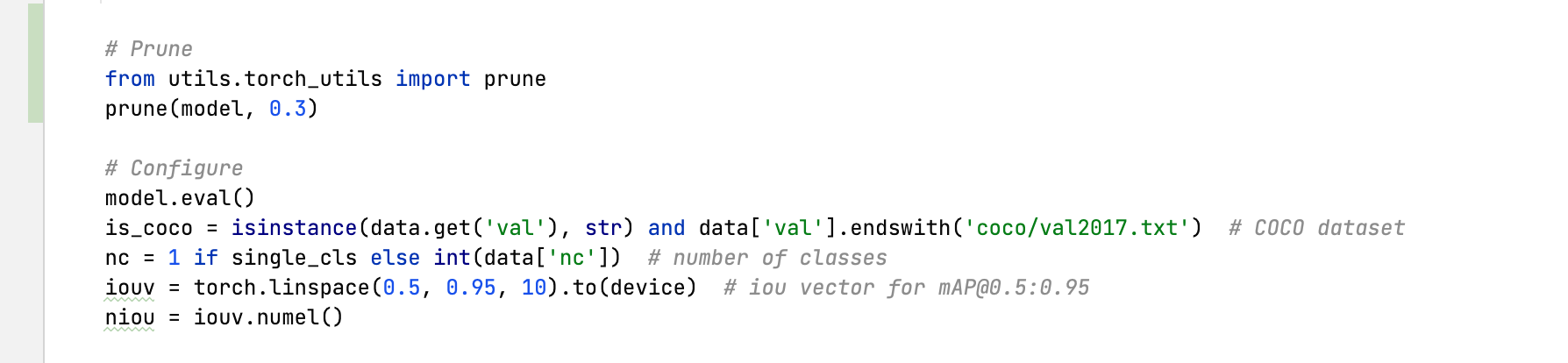# Pruning/Sparsity Tutorial

📚 This guide explains how to apply pruning to YOLOv5 🚀 models. UPDATED 25 September 2022.

## Before You Start

Clone repo and install requirements.txt in a Python>=3.8.0 environment, including PyTorch>=1.8. Models and datasets download automatically from the latest YOLOv5 release.

``````git clone https://github.com/ultralytics/yolov5  # clone
cd yolov5
pip install -r requirements.txt  # install
``````

## Test Normally

Before pruning we want to establish a baseline performance to compare to. This command tests YOLOv5x on COCO val2017 at image size 640 pixels. `yolov5x.pt` is the largest and most accurate model available. Other options are `yolov5s.pt`, `yolov5m.pt` and `yolov5l.pt`, or you own checkpoint from training a custom dataset `./weights/best.pt`. For details on all available models please see our README table.

``````python val.py --weights yolov5x.pt --data coco.yaml --img 640 --half
``````

Output:

``````val: data=/content/yolov5/data/coco.yaml, weights=['yolov5x.pt'], batch_size=32, imgsz=640, conf_thres=0.001, iou_thres=0.65, task=val, device=, workers=8, single_cls=False, augment=False, verbose=False, save_txt=False, save_hybrid=False, save_conf=False, save_json=True, project=runs/val, name=exp, exist_ok=False, half=True, dnn=False
YOLOv5 🚀 v6.0-224-g4c40933 torch 1.10.0+cu111 CUDA:0 (Tesla V100-SXM2-16GB, 16160MiB)

Fusing layers...
Model Summary: 444 layers, 86705005 parameters, 0 gradients
val: Scanning '/content/datasets/coco/val2017.cache' images and labels... 4952 found, 48 missing, 0 empty, 0 corrupt: 100% 5000/5000 [00:00<?, ?it/s]
Class     Images     Labels          P          R     mAP@.5 mAP@.5:.95: 100% 157/157 [01:12<00:00,  2.16it/s]
all       5000      36335      0.732      0.628      0.683      0.496
Speed: 0.1ms pre-process, 5.2ms inference, 1.7ms NMS per image at shape (32, 3, 640, 640)  # <--- base speed

Evaluating pycocotools mAP... saving runs/val/exp2/yolov5x_predictions.json...
...
Average Precision  (AP) @[ IoU=0.50:0.95 | area=   all | maxDets=100 ] = 0.507  # <--- base mAP
Average Precision  (AP) @[ IoU=0.50      | area=   all | maxDets=100 ] = 0.689
Average Precision  (AP) @[ IoU=0.75      | area=   all | maxDets=100 ] = 0.552
Average Precision  (AP) @[ IoU=0.50:0.95 | area= small | maxDets=100 ] = 0.345
Average Precision  (AP) @[ IoU=0.50:0.95 | area=medium | maxDets=100 ] = 0.559
Average Precision  (AP) @[ IoU=0.50:0.95 | area= large | maxDets=100 ] = 0.652
Average Recall     (AR) @[ IoU=0.50:0.95 | area=   all | maxDets=  1 ] = 0.381
Average Recall     (AR) @[ IoU=0.50:0.95 | area=   all | maxDets= 10 ] = 0.630
Average Recall     (AR) @[ IoU=0.50:0.95 | area=   all | maxDets=100 ] = 0.682
Average Recall     (AR) @[ IoU=0.50:0.95 | area= small | maxDets=100 ] = 0.526
Average Recall     (AR) @[ IoU=0.50:0.95 | area=medium | maxDets=100 ] = 0.731
Average Recall     (AR) @[ IoU=0.50:0.95 | area= large | maxDets=100 ] = 0.829
Results saved to runs/val/exp
``````

## Test YOLOv5x on COCO (0.30 sparsity)

We repeat the above test with a pruned model by using the `torch_utils.prune()` command. We update `val.py` to prune YOLOv5x to 0.3 sparsity:30% pruned output:

``````val: data=/content/yolov5/data/coco.yaml, weights=['yolov5x.pt'], batch_size=32, imgsz=640, conf_thres=0.001, iou_thres=0.65, task=val, device=, workers=8, single_cls=False, augment=False, verbose=False, save_txt=False, save_hybrid=False, save_conf=False, save_json=True, project=runs/val, name=exp, exist_ok=False, half=True, dnn=False
YOLOv5 🚀 v6.0-224-g4c40933 torch 1.10.0+cu111 CUDA:0 (Tesla V100-SXM2-16GB, 16160MiB)

Fusing layers...
Model Summary: 444 layers, 86705005 parameters, 0 gradients
Pruning model...  0.3 global sparsity
val: Scanning '/content/datasets/coco/val2017.cache' images and labels... 4952 found, 48 missing, 0 empty, 0 corrupt: 100% 5000/5000 [00:00<?, ?it/s]
Class     Images     Labels          P          R     mAP@.5 mAP@.5:.95: 100% 157/157 [01:11<00:00,  2.19it/s]
all       5000      36335      0.724      0.614      0.671      0.478
Speed: 0.1ms pre-process, 5.2ms inference, 1.7ms NMS per image at shape (32, 3, 640, 640)  # <--- prune mAP

Evaluating pycocotools mAP... saving runs/val/exp3/yolov5x_predictions.json...
...
Average Precision  (AP) @[ IoU=0.50:0.95 | area=   all | maxDets=100 ] = 0.489  # <--- prune mAP
Average Precision  (AP) @[ IoU=0.50      | area=   all | maxDets=100 ] = 0.677
Average Precision  (AP) @[ IoU=0.75      | area=   all | maxDets=100 ] = 0.537
Average Precision  (AP) @[ IoU=0.50:0.95 | area= small | maxDets=100 ] = 0.334
Average Precision  (AP) @[ IoU=0.50:0.95 | area=medium | maxDets=100 ] = 0.542
Average Precision  (AP) @[ IoU=0.50:0.95 | area= large | maxDets=100 ] = 0.635
Average Recall     (AR) @[ IoU=0.50:0.95 | area=   all | maxDets=  1 ] = 0.370
Average Recall     (AR) @[ IoU=0.50:0.95 | area=   all | maxDets= 10 ] = 0.612
Average Recall     (AR) @[ IoU=0.50:0.95 | area=   all | maxDets=100 ] = 0.664
Average Recall     (AR) @[ IoU=0.50:0.95 | area= small | maxDets=100 ] = 0.496
Average Recall     (AR) @[ IoU=0.50:0.95 | area=medium | maxDets=100 ] = 0.722
Average Recall     (AR) @[ IoU=0.50:0.95 | area= large | maxDets=100 ] = 0.803
Results saved to runs/val/exp3
``````

In the results we can observe that we have achieved a sparsity of 30% in our model after pruning, which means that 30% of the model's weight parameters in `nn.Conv2d` layers are equal to 0. Inference time is essentially unchanged, while the model's AP and AR scores a slightly reduced.

## Environments

YOLOv5 is designed to be run in the following up-to-date verified environments (with all dependencies including CUDA/CUDNN, Python and PyTorch preinstalled):

## StatusIf this badge is green, all YOLOv5 GitHub Actions Continuous Integration (CI) tests are currently passing. CI tests verify correct operation of YOLOv5 training, validation, inference, export and benchmarks on macOS, Windows, and Ubuntu every 24 hours and on every commit.

Created 2023-04-21, Updated 2023-08-11
Authors: glenn-jocher (7), sergiuwaxmann (1)